Introduction to Subtraction

 Problem Set 1 Problem Set 2 Problem Set 3Select a problem set using the buttons above, then use your mouse or tab key to select a question. Fill in the blank with the correct answer for each problem. When you have answered all of the questions, ask Charlie how you did.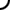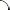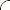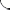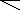Perform each subtraction operation.   5 - 2 = 3 - 8 = 4 - 10 = 10 - 4 = -2 - 6 = -10 - 7 =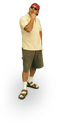Perform each subtraction operation.   5 - 9 = 3 - 1 = 6 - 3 = 3 - 6 = -3 - 7 = -9 - 3 =Perform each subtraction operation.   2 - 7 = 8 - 7 = 4 - 9 = 9 - 4 = -5 - 6 = -7 - 4 =©  2009  LarryPerezWeb Design:  Patrick Quigley